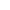﻿ Magic Squares Puzzles ONLINE + worksheets

Page No. 1043

# Magic squares puzzles

Solve magic squares puzzles online. Solving magic squares 3x3. Integer magic squares generator. magic squares pdf. Download printable magic squares worksheet PDF. Math magic squares pdf. Solve magic square puzzles with answers. magic squares online. Play addition squares game online. Generate magic square 3x3 worksheet with answers. Addition magic squares printable. Enjoy magic square sudoku online. Math magic square online. Printable magic square puzzles.

New gameMagic constant:

Fill in the blanks so that the numbers in each row, column and diagonal adds up to the same amount.

PRINT WORKSHEET »

## Magic squares puzzles ONLINESolve magic squares puzzles online. Fill in the missing numbers so the operations in the magic square are correct. A magic square is made up of numbers arranged so that the numbers in each column, row and diagonal produce an equivalent sum. This sum is called "magic constant". Every little square is gonna have an unique value.

### Magic squares puzzles with answers

Press "Check it" to find out if the magic squares puzzle is filled in correctly. The correct answers will be green, wrong results will be red. Press the button "New game" and the new magic squares game will be generated.

## Printable magic squares worksheets PDF

Download printable magic squares in PDF. Everytime a new magic squares worksheet is generated. The first page contains four magic sguares 3x3, the second page contains magic squares puzzles with answers. The magic constants range from 12 to 100.

Click the image bellow and download the free printable magic squares puzzles in PDF:

On these magic squares worksheets, you'll find 4 magic squares puzzles - enough to keep children entertained for a while as they master this fun tasks. You can find more free printable addition worksheets here.

## Magic squares ONLINE

Play magic squares online. Magic squares puzzles are great for teaching addition in a fun and engaging way. These online magic squares enhance not just addition and subtraction, but also problem-solving skills and prepare children for approaching more difficult equations later in their learning journey.

In order to solve these addition squares puzzles, we assume that children already can add and subtract within 100. For practicing addition to 100 check out these addition flash cards, for subtraction up to 100 check out these subtraction flash cards.

You can also try these number crossword puzzles, which also combine addition and subtraction within 100.

## Magic squares 3x3

Our magic squares 3x3 are made up of positive integers arranged so that every vertical, horizontal and diagonal line always adds to the same value. Every little square is gonna have an unique value, it means that these values will not show up again.## Integer magic squares generator

Our magic squares game generate magic squares 3x3. You can choose the range of the magic constant: the range from 12 to 50 is pefect for kids on the first and second grade, the range from 51 to 100 is designed for kids in the second and third grade.

### What are magic squares?

According to Wikipedia magic square is a square array of numbers. This means that they are consecutive numbers. Our magic squares generator generates only addition squares which fulfil the condition, that all the rows, columns and both diagonals equal the same number: the magic constant.

## Magic square game

You can play our magic squares game online or you can print magic square 3x3 worksheet with answers. By giving some of the numbers in the magic square puzzle, children should be able to work out the missing numbers.

### How to solve magic squares with missing numbers?

Start to solve the magic squares game with the rows (or columns or diagonals) that have the most numbers filled in, and think about what number is needed to reach the target magic constant.

Usually, children must first add 2 given numbers and subtract the result from the magic constant. And continue in this way until the magic square puzzle is completely filled.

Once you have filled in all the numbers in the magic square puzzle, check that the numbers in each row, column, and diagonal do indeed add up to the same number. If the numbers do not add up to the same number, you may need to backtrack and try different numbers in the square until you find a solution.